# RD Sharma Solutions for Class 7 Maths Chapter 13 Simple Interest

RD Sharma Solutions for Class 7 Maths Chapter 13 – Simple Interest is the best study material for those students who are finding difficulties in solving problems. Students can refer and download the PDF of RD Sharma Solutions for Class 7 Maths, Chapter 13 Simple Interest from the given links. The solutions to all questions in RD Sharma Solutions for Class 7 are given here in a detailed and step by step way to help the students understand more effectively. Chapter 13 Simple Interest is based on general transactions with interest. RD Sharma Solutions covers all the topic related to it. Some of the important topics of this lesson are listed below.

• Definition of principal
• Definition and meaning of interest
• Formula to calculate the amount
• Definition and meaning of simple interest
• Formula to calculate simple interest
• Verbally related problems on simple interest

## Download the PDF of RD Sharma Solutions For Class 7 Maths Chapter 13 Simple Interest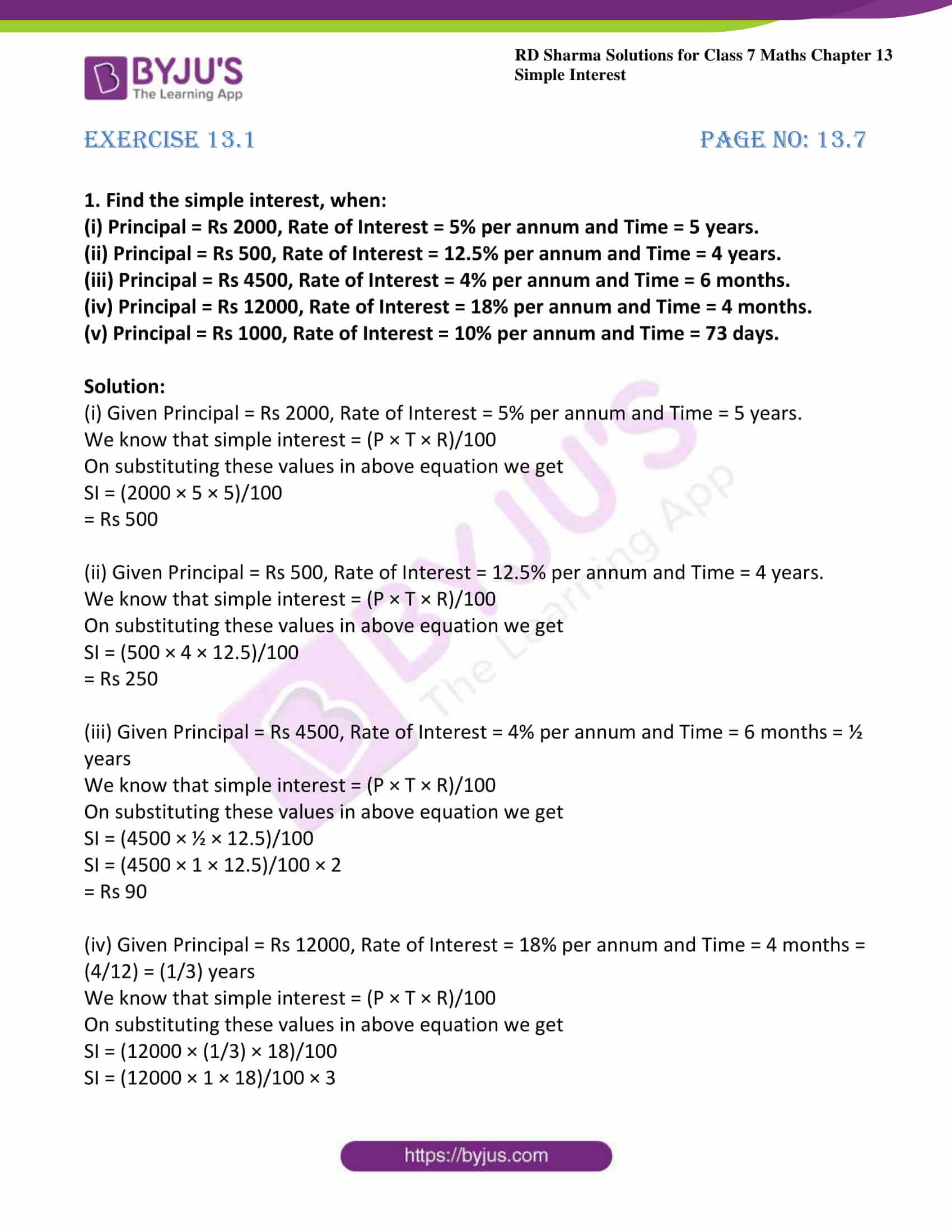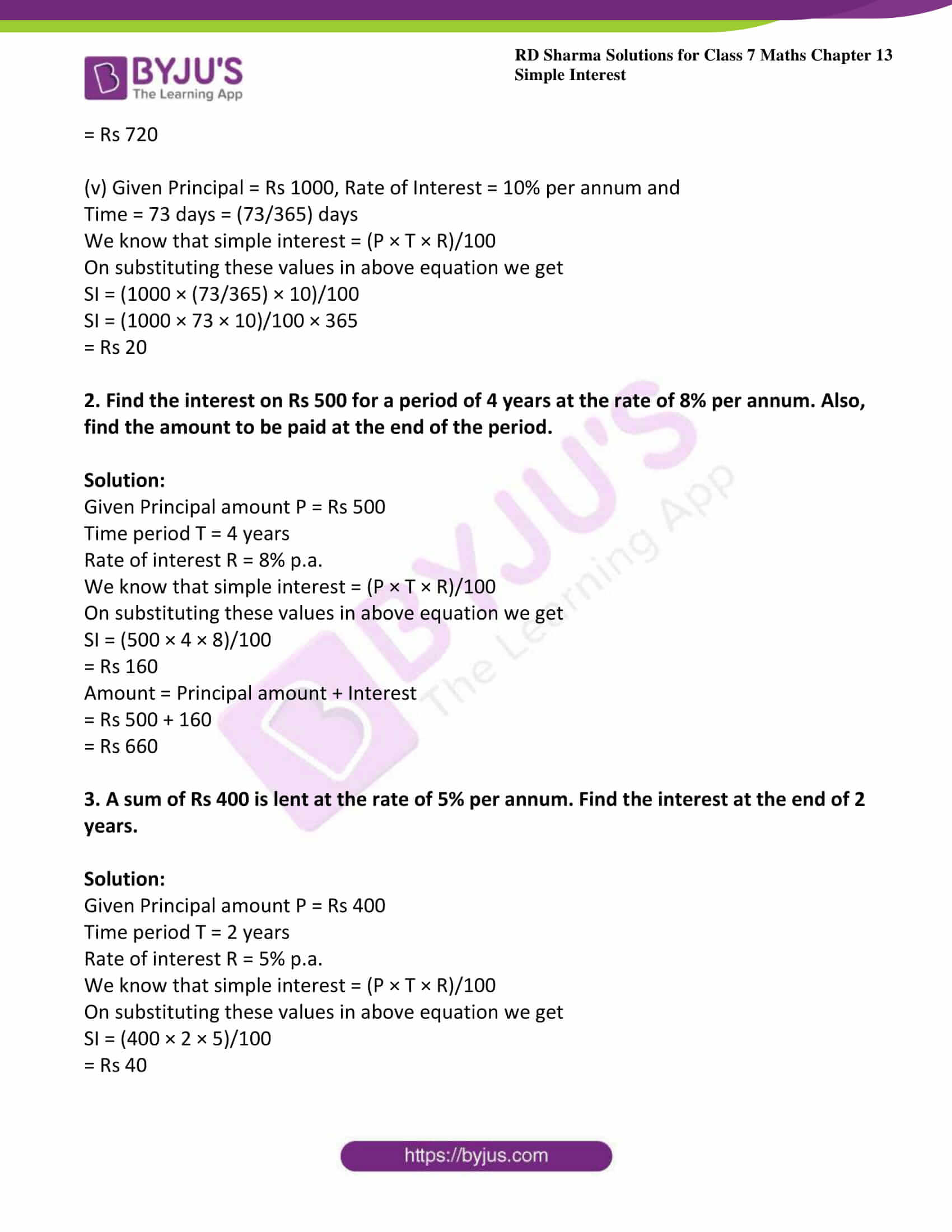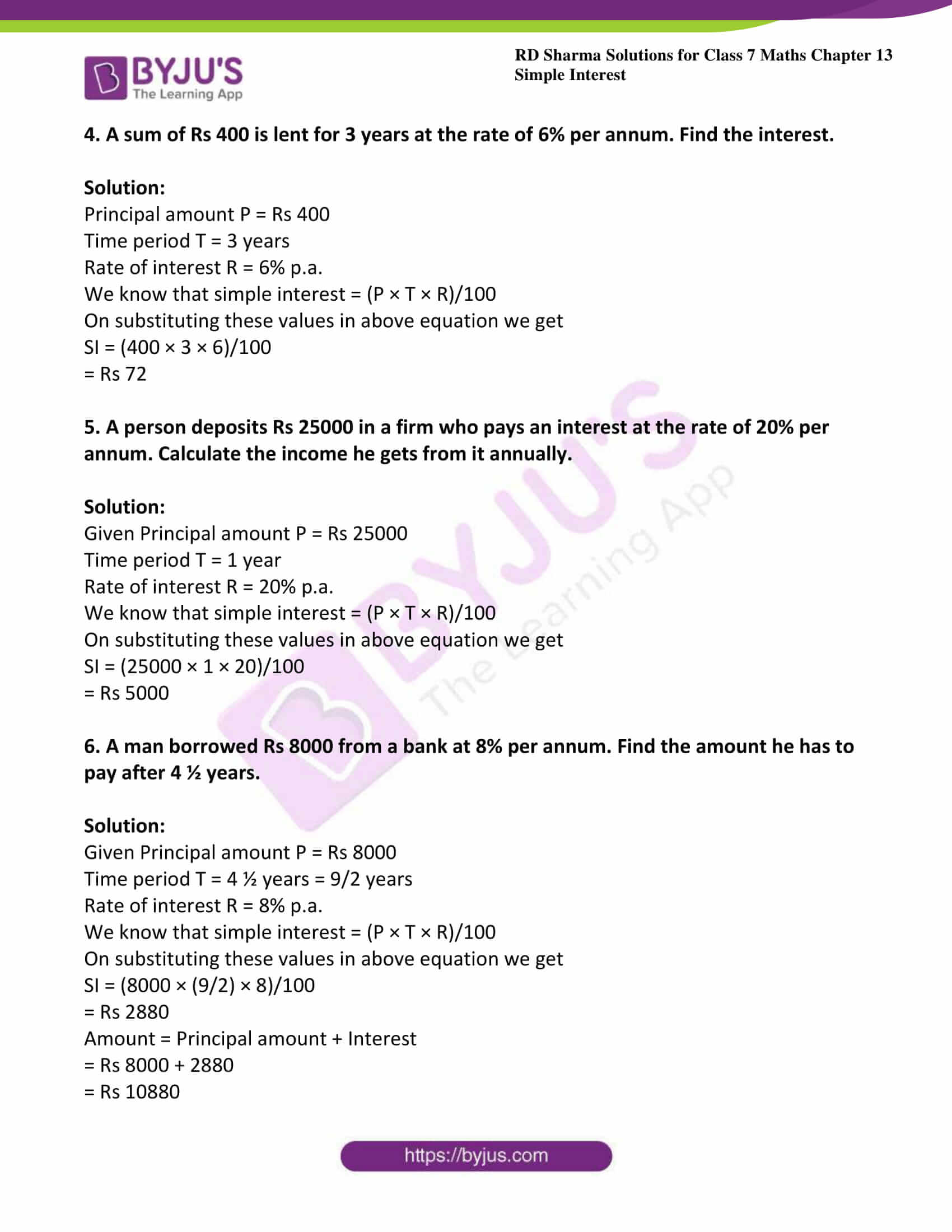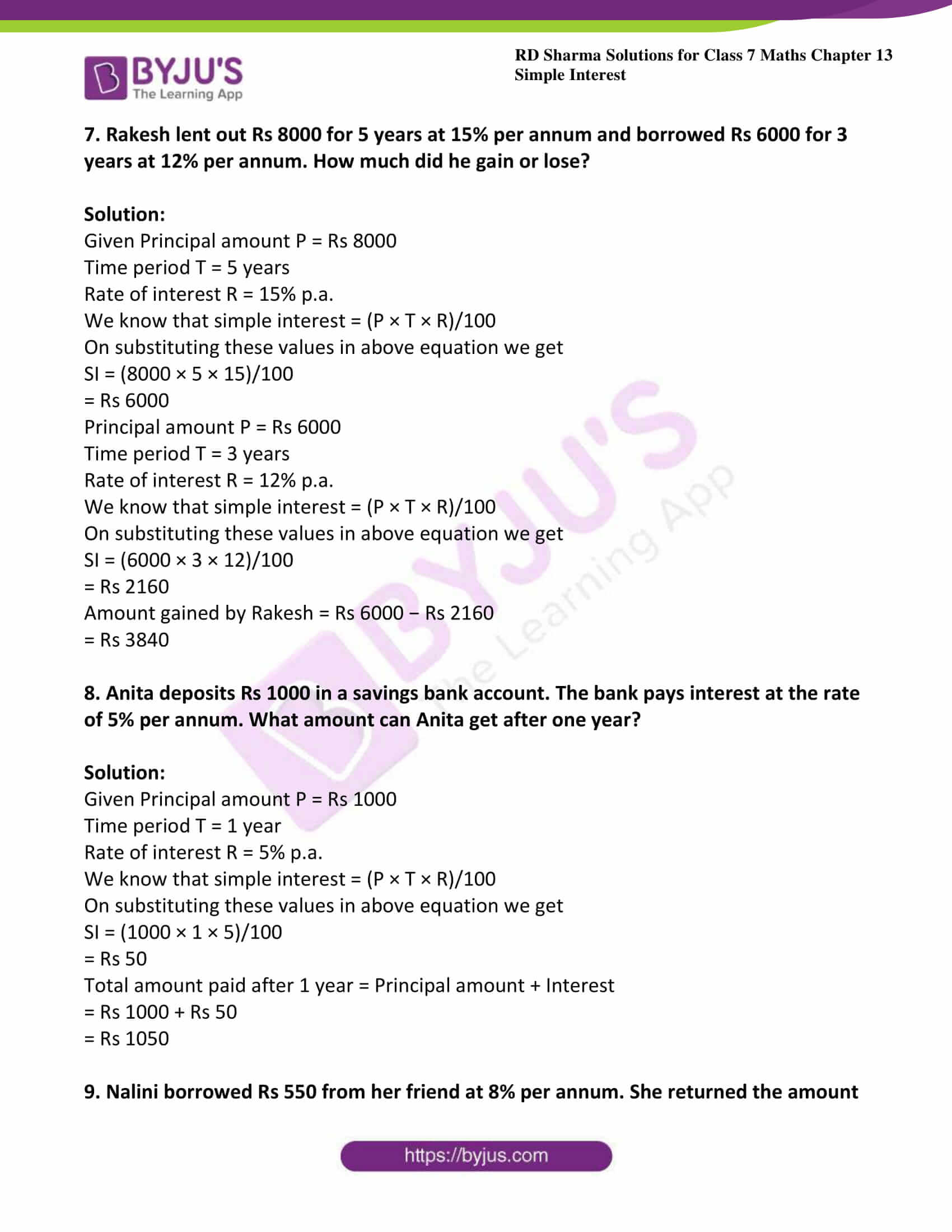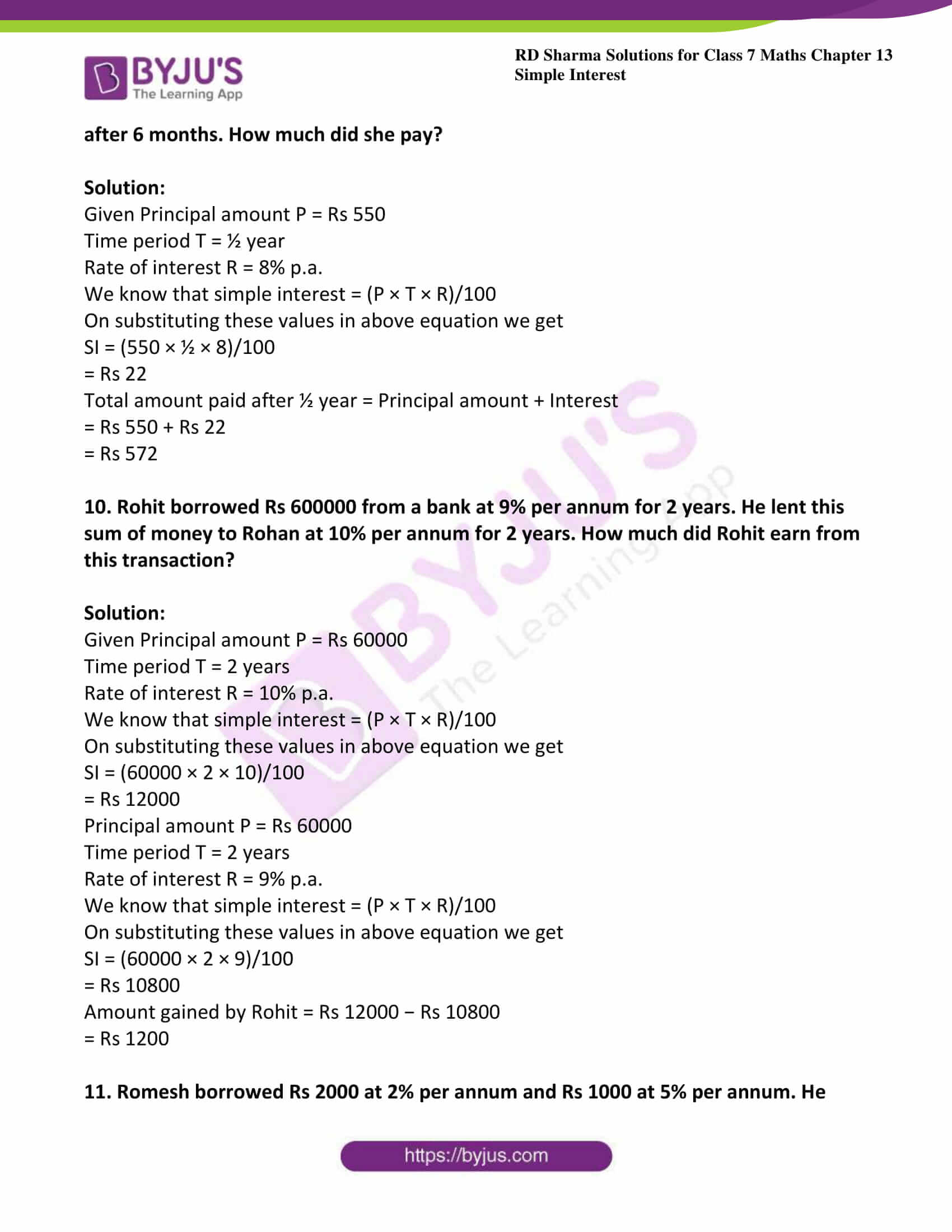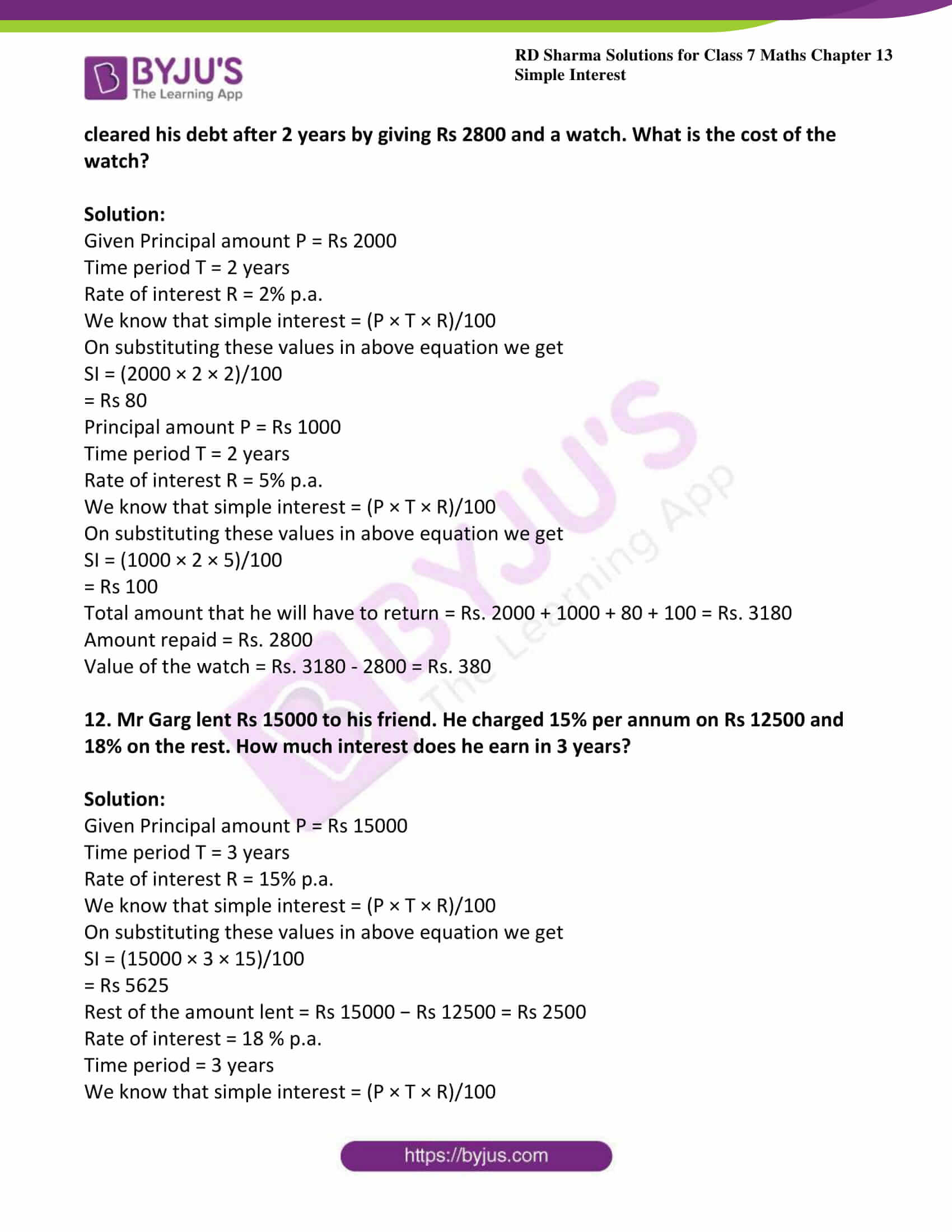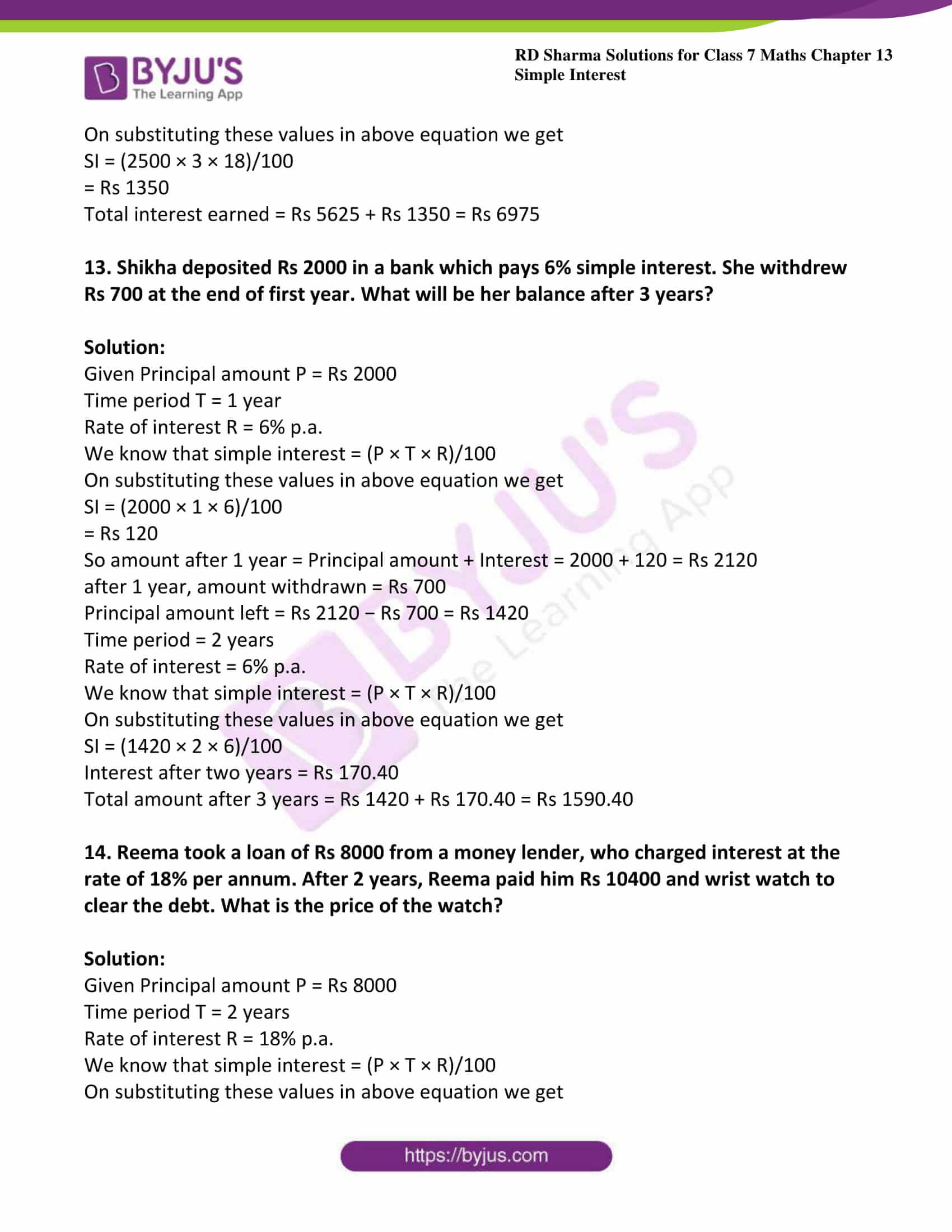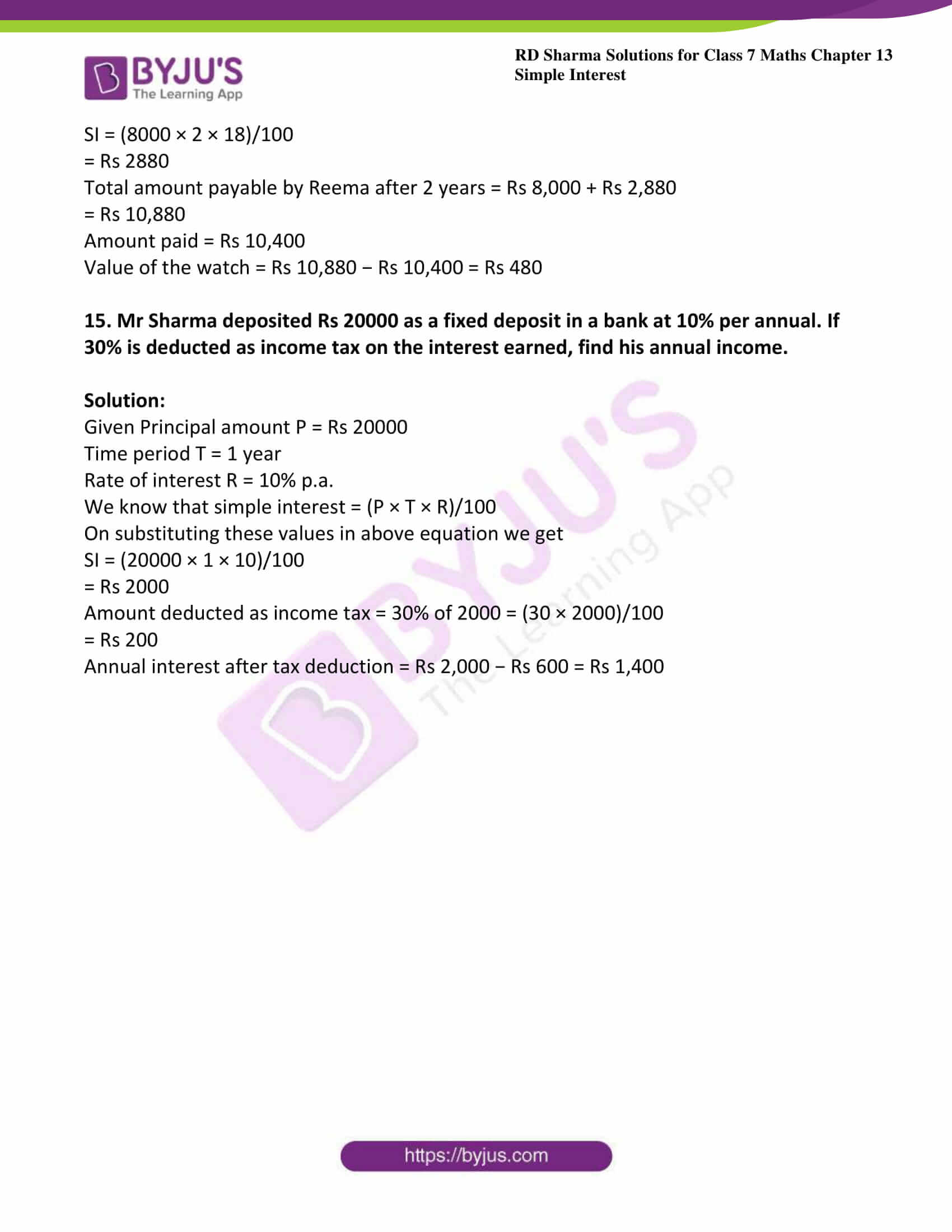### Access answers to Maths RD Sharma Solutions For Class 7 Chapter 13 – Simple Interest

1. Find the simple interest, when:
(i) Principal = Rs 2000, Rate of Interest = 5% per annum and Time = 5 years.
(ii) Principal = Rs 500, Rate of Interest = 12.5% per annum and Time = 4 years.
(iii) Principal = Rs 4500, Rate of Interest = 4% per annum and Time = 6 months.

(iv) Principal = Rs 12000, Rate of Interest = 18% per annum and Time = 4 months.
(v) Principal = Rs 1000, Rate of Interest = 10% per annum and Time = 73 days.

Solution:

(i) Given Principal = Rs 2000, Rate of Interest = 5% per annum and Time = 5 years.

We know that simple interest = (P × T × R)/100

On substituting these values in above equation we get

SI = (2000 × 5 × 5)/100

= Rs 500

(ii) Given Principal = Rs 500, Rate of Interest = 12.5% per annum and Time = 4 years.

We know that simple interest = (P × T × R)/100

On substituting these values in above equation we get

SI = (500 × 4 × 12.5)/100

= Rs 250

(iii) Given Principal = Rs 4500, Rate of Interest = 4% per annum and Time = 6 months = ½ years

We know that simple interest = (P × T × R)/100

On substituting these values in above equation we get

SI = (4500 × ½ × 12.5)/100

SI = (4500 × 1 × 12.5)/100 × 2

= Rs 90

(iv) Given Principal = Rs 12000, Rate of Interest = 18% per annum and Time = 4 months = (4/12) = (1/3) years

We know that simple interest = (P × T × R)/100

On substituting these values in above equation we get

SI = (12000 × (1/3) × 18)/100

SI = (12000 × 1 × 18)/100 × 3

= Rs 720

(v) Given Principal = Rs 1000, Rate of Interest = 10% per annum and

Time = 73 days = (73/365) days

We know that simple interest = (P × T × R)/100

On substituting these values in above equation we get

SI = (1000 × (73/365) × 10)/100

SI = (1000 × 73 × 10)/100 × 365

= Rs 20

2. Find the interest on Rs 500 for a period of 4 years at the rate of 8% per annum. Also, find the amount to be paid at the end of the period.

Solution:

Given Principal amount P = Rs 500

Time period T = 4 years

Rate of interest R = 8% p.a.

We know that simple interest = (P × T × R)/100

On substituting these values in above equation we get

SI = (500 × 4 × 8)/100

= Rs 160

Amount = Principal amount + Interest

= Rs 500 + 160

= Rs 660

3. A sum of Rs 400 is lent at the rate of 5% per annum. Find the interest at the end of 2 years.

Solution:

Given Principal amount P = Rs 400

Time period T = 2 years

Rate of interest R = 5% p.a.

We know that simple interest = (P × T × R)/100

On substituting these values in above equation we get

SI = (400 × 2 × 5)/100

= Rs 40

4. A sum of Rs 400 is lent for 3 years at the rate of 6% per annum. Find the interest.

Solution:

Principal amount P = Rs 400

Time period T = 3 years

Rate of interest R = 6% p.a.

We know that simple interest = (P × T × R)/100

On substituting these values in above equation we get

SI = (400 × 3 × 6)/100

= Rs 72

5. A person deposits Rs 25000 in a firm who pays an interest at the rate of 20% per annum. Calculate the income he gets from it annually.

Solution:

Given Principal amount P = Rs 25000

Time period T = 1 year

Rate of interest R = 20% p.a.

We know that simple interest = (P × T × R)/100

On substituting these values in above equation we get

SI = (25000 × 1 × 20)/100

= Rs 5000

6. A man borrowed Rs 8000 from a bank at 8% per annum. Find the amount he has to pay after 4 ½ years.

Solution:

Given Principal amount P = Rs 8000

Time period T = 4 ½ years = 9/2 years

Rate of interest R = 8% p.a.

We know that simple interest = (P × T × R)/100

On substituting these values in above equation we get

SI = (8000 × (9/2) × 8)/100

= Rs 2880

Amount = Principal amount + Interest

= Rs 8000 + 2880

= Rs 10880

7. Rakesh lent out Rs 8000 for 5 years at 15% per annum and borrowed Rs 6000 for 3 years at 12% per annum. How much did he gain or lose?

Solution:

Given Principal amount P = Rs 8000

Time period T = 5 years

Rate of interest R = 15% p.a.

We know that simple interest = (P × T × R)/100

On substituting these values in above equation we get

SI = (8000 × 5 × 15)/100

= Rs 6000

Principal amount P = Rs 6000

Time period T = 3 years

Rate of interest R = 12% p.a.

We know that simple interest = (P × T × R)/100

On substituting these values in above equation we get

SI = (6000 × 3 × 12)/100

= Rs 2160

Amount gained by Rakesh = Rs 6000 − Rs 2160

= Rs 3840

8. Anita deposits Rs 1000 in a savings bank account. The bank pays interest at the rate of 5% per annum. What amount can Anita get after one year?

Solution:

Given Principal amount P = Rs 1000

Time period T = 1 year

Rate of interest R = 5% p.a.

We know that simple interest = (P × T × R)/100

On substituting these values in above equation we get

SI = (1000 × 1 × 5)/100

= Rs 50

Total amount paid after 1 year = Principal amount + Interest

= Rs 1000 + Rs 50

= Rs 1050

9. Nalini borrowed Rs 550 from her friend at 8% per annum. She returned the amount after 6 months. How much did she pay?

Solution:

Given Principal amount P = Rs 550

Time period T = ½ year

Rate of interest R = 8% p.a.

We know that simple interest = (P × T × R)/100

On substituting these values in above equation we get

SI = (550 × ½ × 8)/100

= Rs 22

Total amount paid after ½ year = Principal amount + Interest

= Rs 550 + Rs 22

= Rs 572

10. Rohit borrowed Rs 600000 from a bank at 9% per annum for 2 years. He lent this sum of money to Rohan at 10% per annum for 2 years. How much did Rohit earn from this transaction?

Solution:

Given Principal amount P = Rs 60000

Time period T = 2 years

Rate of interest R = 10% p.a.

We know that simple interest = (P × T × R)/100

On substituting these values in above equation we get

SI = (60000 × 2 × 10)/100

= Rs 12000

Principal amount P = Rs 60000

Time period T = 2 years

Rate of interest R = 9% p.a.

We know that simple interest = (P × T × R)/100

On substituting these values in above equation we get

SI = (60000 × 2 × 9)/100

= Rs 10800

Amount gained by Rohit = Rs 12000 − Rs 10800

= Rs 1200

11. Romesh borrowed Rs 2000 at 2% per annum and Rs 1000 at 5% per annum. He cleared his debt after 2 years by giving Rs 2800 and a watch. What is the cost of the watch?

Solution:

Given Principal amount P = Rs 2000

Time period T = 2 years

Rate of interest R = 2% p.a.

We know that simple interest = (P × T × R)/100

On substituting these values in above equation we get

SI = (2000 × 2 × 2)/100

= Rs 80

Principal amount P = Rs 1000

Time period T = 2 years

Rate of interest R = 5% p.a.

We know that simple interest = (P × T × R)/100

On substituting these values in above equation we get

SI = (1000 × 2 × 5)/100

= Rs 100

Total amount that he will have to return = Rs. 2000 + 1000 + 80 + 100 = Rs. 3180

Amount repaid = Rs. 2800

Value of the watch = Rs. 3180 – 2800 = Rs. 380

12. Mr Garg lent Rs 15000 to his friend. He charged 15% per annum on Rs 12500 and 18% on the rest. How much interest does he earn in 3 years?

Solution:

Given Principal amount P = Rs 15000

Time period T = 3 years

Rate of interest R = 15% p.a.

We know that simple interest = (P × T × R)/100

On substituting these values in above equation we get

SI = (15000 × 3 × 15)/100

= Rs 5625

Rest of the amount lent = Rs 15000 − Rs 12500 = Rs 2500

Rate of interest = 18 % p.a.

Time period = 3 years

We know that simple interest = (P × T × R)/100

On substituting these values in above equation we get

SI = (2500 × 3 × 18)/100

= Rs 1350

Total interest earned = Rs 5625 + Rs 1350 = Rs 6975

13. Shikha deposited Rs 2000 in a bank which pays 6% simple interest. She withdrew Rs 700 at the end of first year. What will be her balance after 3 years?

Solution:

Given Principal amount P = Rs 2000

Time period T = 1 year

Rate of interest R = 6% p.a.

We know that simple interest = (P × T × R)/100

On substituting these values in above equation we get

SI = (2000 × 1 × 6)/100

= Rs 120

So amount after 1 year = Principal amount + Interest = 2000 + 120 = Rs 2120

after 1 year, amount withdrawn = Rs 700

Principal amount left = Rs 2120 − Rs 700 = Rs 1420

Time period = 2 years

Rate of interest = 6% p.a.

We know that simple interest = (P × T × R)/100

On substituting these values in above equation we get

SI = (1420 × 2 × 6)/100

Interest after two years = Rs 170.40

Total amount after 3 years = Rs 1420 + Rs 170.40 = Rs 1590.40

14. Reema took a loan of Rs 8000 from a money lender, who charged interest at the rate of 18% per annum. After 2 years, Reema paid him Rs 10400 and wrist watch to clear the debt. What is the price of the watch?

Solution:

Given Principal amount P = Rs 8000

Time period T = 2 years

Rate of interest R = 18% p.a.

We know that simple interest = (P × T × R)/100

On substituting these values in above equation we get

SI = (8000 × 2 × 18)/100

= Rs 2880

Total amount payable by Reema after 2 years = Rs 8,000 + Rs 2,880

= Rs 10,880

Amount paid = Rs 10,400

Value of the watch = Rs 10,880 − Rs 10,400 = Rs 480

15. Mr Sharma deposited Rs 20000 as a fixed deposit in a bank at 10% per annual. If 30% is deducted as income tax on the interest earned, find his annual income.

Solution:

Given Principal amount P = Rs 20000

Time period T = 1 year

Rate of interest R = 10% p.a.

We know that simple interest = (P × T × R)/100

On substituting these values in above equation we get

SI = (20000 × 1 × 10)/100

= Rs 2000

Amount deducted as income tax = 30% of 2000 = (30 × 2000)/100

= Rs 200

Annual interest after tax deduction = Rs 2,000 − Rs 600 = Rs 1,400